Share this: Google+

## Symmetric Matrix using Excel

Suppose we have a integer matrix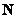, to get symmetric matrix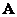from, we multiply matrixby its transpose: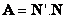or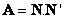. Here is how to do it in Excel. You may download the spreadsheet example here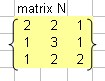1. Type any matrix 3 by 3. Suppose our matrix is like this figure on the right
2. Note that optionally you can give name to the matrix using menu Insert-Name-Define matrix_N , then press OK button.
3. In other cell, type =TRANSPOSE( matrix_N ), where the array is the 3 by 3 matrix N. Then press enter key.
4. Don't worry about the result yet. Starting from the cell with Transpose formula, highlight 3 by 3 cells. Press F2, and it will be appear like the following figure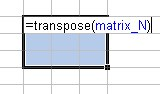. Then press CTRL-SHIFT-ENTER together. You get the transpose matrix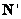. Optionally you may give name matrix_N1 to the transpose matrix by using procedure in step 2 above.
5. Now you can get the symmetric matrix by multiplying multiply matrixby its transpose. In a new empty cell type = MMULT(matrix_N1, matrix_N) and then press enter key.
6. Starting with the cell with MMult formula, highlight 3 by 3 cells. Press F2, and it will be appear like the following figure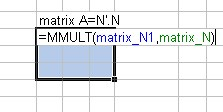Then press CTRL-SHIFT-ENTER together you get the symmetric matrixas shown in the figure below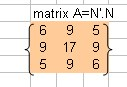Preferable reference for this tutorial is

Teknomo, Kardi (2015) How do we get symmetric matrix ?. https:\\people.revoledu.com\kardi\ tutorial\Excel\SymmetricMatrix.html